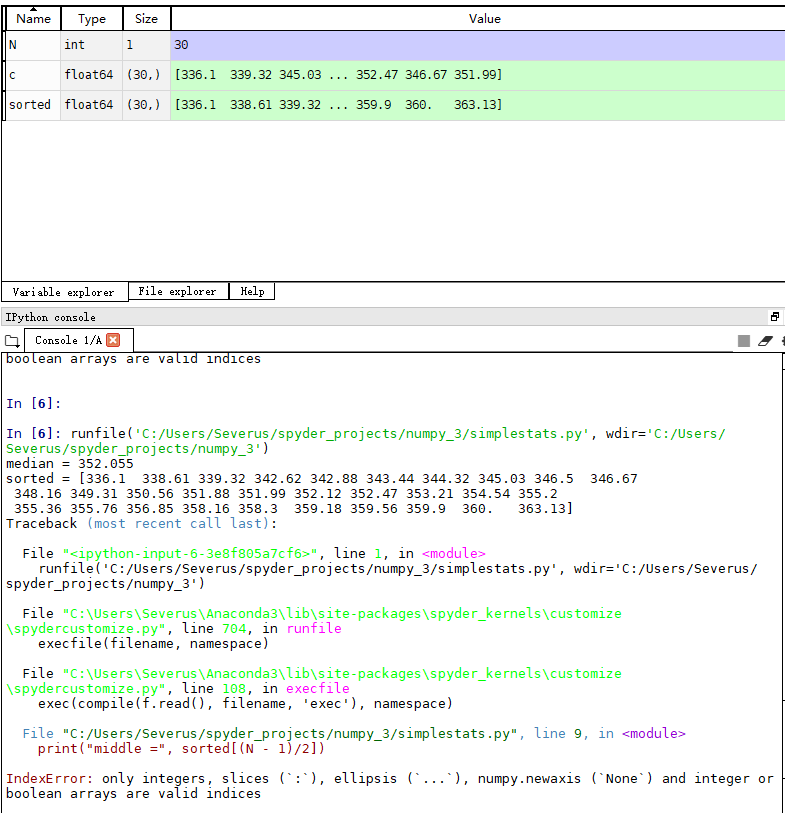2019-09-30 22:41

# sorted 函数bug，有没有大神帮忙指点下这是什么错误

import numpy as np

c = np.loadtxt('data.csv', delimiter = ',', usecols = (6, ), unpack = True)
print ("median =", np.median(c))
sorted = np.msort(c)
print ("sorted =", sorted)

N = len(c)
print("middle =", sorted[(N - 1)/2])
print("average middle =", (sorted[N / 2] + sorted[(N - 1) / 2]) / 2)

print("variance =", np.var(c))
print("variance from definition =", np.mean((c - c.mean()) ** 2))``````
``````
• 写回答
• 好问题 提建议
• 追加酬金
• 关注问题
• 邀请回答